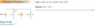# Can this circuit be solved without nodal analysis?

• Engineering
Anti Hydrogen
Homework Statement:
The book hasn't presented the nodal analysis before this problem, but i can't tell what technique to use in this situation
Relevant Equations:
any hint?
help will be appreciated

#### Attachments

•Untitled.png
9 KB · Views: 192

Gold Member
Can you show us your attempt at a solution? Can you write down any equations to represent the relationships shown in the schematic?
Sometimes when you don't know where to start, just writing down what you can deduce from the circuit can get you started.
I'm not sure how to answer your question about nodal analysis. My instinct says; "yes, absolutely, you can usually solve simple circuits without formal nodal analysis". However, virtually all circuit analysis is nodal analysis in disguise or with short-cuts.

Anti Hydrogen
Can you show us your attempt at a solution? Can you write down any equations to represent the relationships shown in the schematic?
Sometimes when you don't know where to start, just writing down what you can deduce from the circuit can get you started.
I'm not sure how to answer your question about nodal analysis. My instinct says; "yes, absolutely, you can usually solve simple circuits without formal nodal analysis". However, virtually all circuit analysis is nodal analysis in disguise or with short-cuts.
The book only presented the kirchhoff's laws and the ohm's law in that chapter.
The circuit clearly has one node (and the reference node); I applied de KCL to the top node but it gave an equation with 2 unknown currents

Gold Member
The circuit clearly has one node (and the reference node); I applied de KCL to the top node
That's a good start. What else do you know about the circuit? You have 1 equation with 2 unknowns, so either it can't be solved uniquely, or you need more equations.

Gold Member
Do you think the value of the resistors would effect the solution? If, so, where do they appear in your equations?

Anti Hydrogen
or you need more equations.
Yes, in order to have another equation, the circuit would need another node, I think

Gold Member
Yes, in order to have another equation, the circuit would need another node, I think
No.
Suppose I told you the value of Io. Could you solve for all of the other voltages and currents in the circuit. What equations would you use to do that?

Anti Hydrogen
Do you think the value of the resistors would effect the solution?
Yes they do undoubtedly affect the solution, the problem is asking for the voltage across the left resistor which is obtained by ohm's law V=IR

Anti Hydrogen
No.
Suppose I told you the value of Io. Could you solve for all of the other voltages and currents in the circuit. What equations would you use to do that?
With that information given, the other variables would be obtained by ohm's law, simple

Mentor
I'm rather dubious of the supplied answers. If ##i_o = 10 A## then already with the controlled source and the current through the 2 Ω resistor you've exceeded the current being supplied to the node (15 A).

••DaveE and Anti Hydrogen
Gold Member
I'm rather dubious of the supplied answers. If ##i_o = 10 A## then already with the controlled source and the current through the 2 Ω resistor you've exceeded the current being supplied to the node (15 A).
Yes, they are wrong. It never occurred to me to put the numbers into my equations, LOL. I ALWAYS leave that as the last step.
The correct answers are (90/13)A and (180/13)V.

•Anti Hydrogen
Gold Member
With that information given, the other variables would be obtained by ohm's law, simple
KCL is almost always used with KVL and ohms law. Sometimes the circuits are so simple that you don't recognize it though. For example, if you were to say it's obvious that the voltage across the 2 resistors in this circuit is the same value, you would actually be applying KVL to do that. If you combine that with ohms law you can get a relationship between the currents through those resistors.

Anti Hydrogen
Yes, they are wrong. It never occurred to me to put the numbers into my equations, LOL. I ALWAYS leave that as the last step.
The correct answers are (90/13)A and (180/13)V.
I got the same answers after some calculation (I had to use nodal analysis) and yes, the book is wrong, thanks everyone for helping

•DaveE and berkeman
Anti Hydrogen
i use fundamentals of electric circuits by sadiku 6th edition by the way

Mentor
I got the same answers after some calculation (I had to use nodal analysis) and yes, the book is wrong, thanks everyone for helping
Nodal analysis when there is only one essential node is just KCL in its basic form. Yes, you also need Ohm's law to complete the analysis, but that's just another "primitive" circuit law.

So, well done!

•Anti Hydrogen
Mentor
i use fundamentals of electric circuits by sadiku 6th edition by the way
Good to know. We (PF Homework Helpers et al) can keep an eye out for possible errors when other members say that their problem comes from this source.

••berkeman and Anti Hydrogen